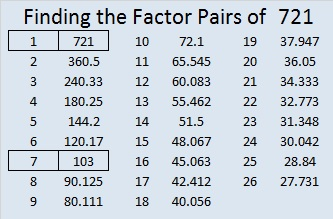# 721 Merry Christmas, Everybody!

It isn’t difficult to see that 721 is divisible by 7.

• 721 is a composite number.
• Prime factorization: 721 = 7 x 103
• The exponents in the prime factorization are 1 and 1. Adding one to each and multiplying we get (1 + 1)(1 + 1) = 2 x 2 = 4. Therefore 721 has exactly 4 factors.
• Factors of 721: 1, 7, 103, 721
• Factor pairs: 721 = 1 x 721 or 7 x 103
• 721 has no square factors that allow its square root to be simplified. √721 ≈ 26.85144.Merry Christmas everybody! Today’s puzzle is not too hard and not too easy so enjoy solving it during your leisure hours today.Print the puzzles or type the solution on this excel file: 10 Factors 2015-12-21

——————————————————————————————

Here is a little more about the number 721:

721 is the sum of the nine prime numbers from 61 to 101.

Stetson.edu informs us that 721 can be expressed as the difference of two cubes two different ways, and is the smallest number that can make that claim. The two ways were fairly easy to find:

• 9^3 – 2^3 = 721
• 16^3 – 15^3 = 721

Because it is equal to the difference of the 16th and 15th cubes, 721 is the 16th centered hexagonal number.

And 721 is a palindrome in two bases:

• 1G1 in base 20 (G = 16 base 10); note that 1(400) + 16(20) + 1(1) = 721.
• 161 base 24; note that 1(24²) + 6(24) + 1(1) = 721.

——————————————————————————————